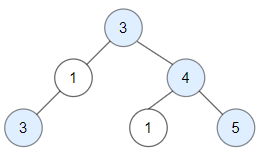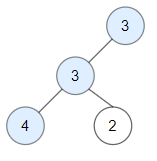1448. Count Good Nodes in Binary Tree

#### QUESTION:

Given a binary tree root, a node X in the tree is named good if in the path from root to X there are no nodes with a value greater than X.

Return the number of good nodes in the binary tree.

Example 1:``````Input: root = [3,1,4,3,null,1,5]
Output: 4
Explanation: Nodes in blue are good.
Root Node (3) is always a good node.
Node 4 -> (3,4) is the maximum value in the path starting from the root.
Node 5 -> (3,4,5) is the maximum value in the path
Node 3 -> (3,1,3) is the maximum value in the path.
``````

Example 2:``````Input: root = [3,3,null,4,2]
Output: 3
Explanation: Node 2 -> (3, 3, 2) is not good, because "3" is higher than it.
``````

Example 3:

``````Input: root = 
Output: 1
Explanation: Root is considered as good.
``````

Constraints:

``````The number of nodes in the binary tree is in the range [1, 10^5].
Each node's value is between [-10^4, 10^4].
``````

#### SOLUTION:

``````class Solution {
func goodNodes(_ root: TreeNode?) -> Int {
var arr:[Int] = []
return goodNodesHelper(root, arr: &arr)
}

func goodNodesHelper(_ root: TreeNode?, arr: inout [Int]) -> Int {
arr.append(root!.val)
var result:Int = 0
if arr.max() == root!.val {
result = 1
}
var left:Int = 0
if ((root?.left) != nil) {
left = goodNodesHelper(root?.left, arr: &arr)
}
var right:Int = 0
if ((root?.right) != nil) {
right = goodNodesHelper(root?.right, arr: &arr)
}
arr.removeLast()
return result + left + right
}
}
``````